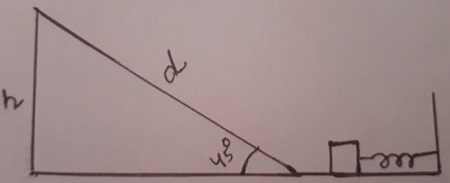A 0.76 kg block is shot horizontally from a spring, as in the example above, and travels 0.516 m...

Question:

A 0.76 kg block is shot horizontally from a spring, as in the example above, and travels 0.516 m up a long a frictionless ramp before coming to rest and sliding back down. If the ramp makes an angle of 45.0{eq}^{\circ} {/eq} with respect to the horizontal, and the spring originally was compressed by 0.16 m, find the spring constant. N/m

Energy Conservation Principle in Spring Block System:

The loss in elastic potential energy of a block is equal to the gain in gravitational potential energy, provided the change in kinetic energy is zero.The elastic potential energy of a spring constant k = {eq}\displaystyle \frac{1}{2}kx^{2} {/eq}

Given, Mass of block (m) = 0.76 kg.

Distance traveled by the block along the ramp (d) = 0.516 m

Angle of inclination {eq}\displaystyle (\theta)= 45^{0} {/eq}

Compression of the spring (x) = 0.16 m.

Now according to Energy conservation principle

loss in elastic potential energy of a block= gain in gravitational potential energy

{eq}\displaystyle \frac{1}{2}kx^{2}=mgh {/eq} -------(1)

Here, h= vertical height reached by the block = {eq}\displaystyle d sin 45^{0} {/eq}

{eq}\displaystyle = 0.516\times 0.7=0.3612 m {/eq}

and g = acceleration due to gravity = {eq}\displaystyle \frac{m}{s^{2}} {/eq}

Now from equation (1)

{eq}\displaystyle kx^{2}=2mgh {/eq}

{eq}\displaystyle k=\frac{2mgh}{x^{2}} {/eq}

{eq}\displaystyle k= \frac{2\times 0.76\times 9.8\times 0.3612}{(0.16)^{2}} = \frac{5.380}{0.0256} = \boxed{210.15 N/m} {/eq}